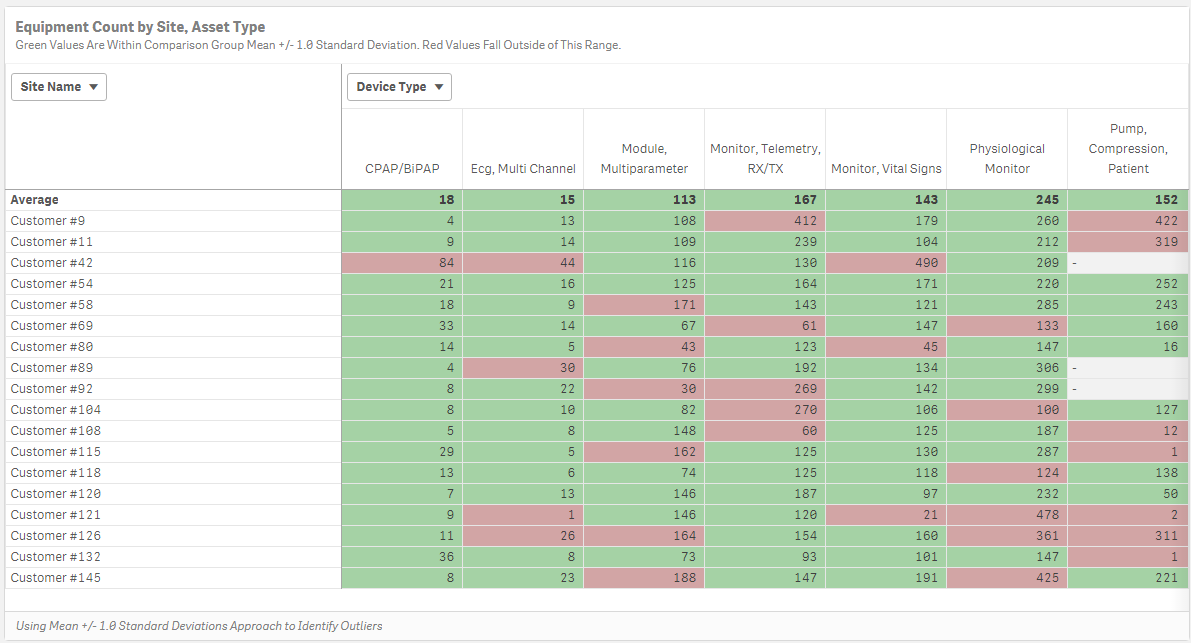# New to Qlik Sense

If you’re new to Qlik Sense, start with this Discussion Board and get up-to-speed quickly.

Announcements
QlikWorld 2023, a live, in-person thrill ride. Save \$300 before February 6: REGISTER NOW!
cancel
Showing results for
Did you mean:Contributor III

## Set Analysis Help Needed

Hi!

I am working on creating a set analysis expression and need some help or pointers from the experts.

1. I calculate a number, using the following expression: =avg(aggr(sum(IB_COUNTER),[%ENCRYPTED_NAME], [%IB_DEVICE_TYPE]))

2. What I wan to do next is calculate a MAX((aggr(sum(IB_COUNTER),[%ENCRYPTED_NAME], [%IB_DEVICE_TYPE])), but only for the values that meet the following criteria:

=fabs(Sum(TOTAL<[%IB_DEVICE_TYPE]> IB_COUNTER) / COUNT(TOTAL<[%IB_DEVICE_TYPE]> DISTINCT [%ENCRYPTED_NAME]) - avg(aggr(sum(IB_COUNTER),[%ENCRYPTED_NAME], [%IB_DEVICE_TYPE]))) > \$(vOutliersThreshold) * Stdev(TOTAL<[%IB_DEVICE_TYPE]> Aggr(Sum(IB_COUNTER) ,[%IB_DEVICE_TYPE],[%ENCRYPTED_NAME]))

I think this could be done using indirect set analysis, but I am struggling with constructing the right expression.

GREATLY APPRECIATE ANY HELP!!

1 Solution

Accepted SolutionsMVP

Try this

If(Dimensionality() = 1, sum([Device Count]),

avg(aggr(if(fabs(Sum(TOTAL<DeviceType> [Device Count]) / COUNT(TOTAL<DeviceType> DISTINCT Customer) - avg(aggr(sum([Device Count]),Customer, DeviceType)))

<= 1*Stdev(TOTAL<DeviceType> Aggr(Sum([Device Count]) ,DeviceType,Customer)), sum([Device Count])),Customer, DeviceType)))10 RepliesMVP

Would you be able to share a sample or some raw data and explain the expected output out of this sample?Contributor III
Author

Sunny,I have color coded cells RED, when the value falls outside of Avg Value (first row) + 1 Std. Dev. These are outliers. Now, I would like to calculate the average value, for each Device Type, but only for cells that are green.MVP

May be this

=Avg(Aggr(

If(fabs(Sum(TOTAL<[%IB_DEVICE_TYPE]> IB_COUNTER) / COUNT(TOTAL<[%IB_DEVICE_TYPE]> DISTINCT [%ENCRYPTED_NAME]) - avg(aggr(sum(IB_COUNTER),[%ENCRYPTED_NAME], [%IB_DEVICE_TYPE]))) > \$(vOutliersThreshold) * Stdev(TOTAL<[%IB_DEVICE_TYPE]> Aggr(Sum(IB_COUNTER) ,[%IB_DEVICE_TYPE],[%ENCRYPTED_NAME])),

Sum(IB_COUNTER), [%ENCRYPTED_NAME], [%IB_DEVICE_TYPE]))Contributor III
Author

Sunny, does not work.If you look at the image attached, value returned for the first Device Type (CPAP, BiPAP) should be 14 (average of all values in the CPAP/BiPAP column, excluding 84, the outlier).MVP

What does the above give you?Contributor III
Author

Error. It does not return anythingMVP

Would you be able to share a sample qvf file?Contributor III
Author

here it isMVP

Try this

If(Dimensionality() = 1, sum([Device Count]),

avg(aggr(if(fabs(Sum(TOTAL<DeviceType> [Device Count]) / COUNT(TOTAL<DeviceType> DISTINCT Customer) - avg(aggr(sum([Device Count]),Customer, DeviceType)))

<= 1*Stdev(TOTAL<DeviceType> Aggr(Sum([Device Count]) ,DeviceType,Customer)), sum([Device Count])),Customer, DeviceType)))Tags
Community Browser